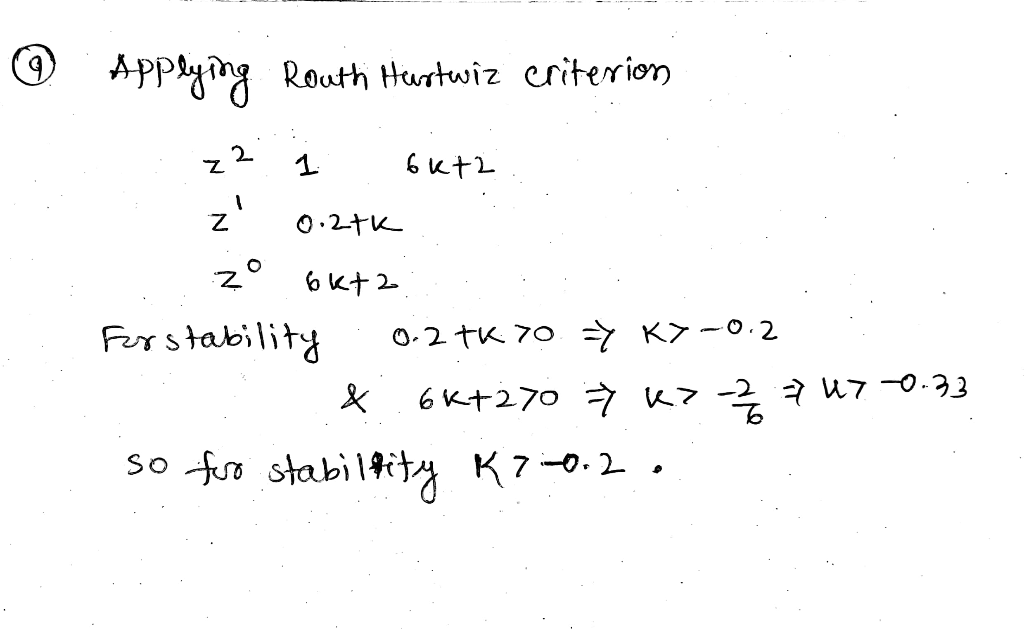Homework Help Question & Answers

Use rlocus in MATLAB to plot the root locus for a closed loop control system with the plant trans...Use rlocus in MATLAB to plot the root locus for a closed loop control system with the plant transfer function 8. z 2 2)2-0.1z +0.06 For what value of k is the closed loop system stable? 9. The characteristic equation for a control system is given as z2(0.2 +k)z 6k +2-0 Use Routh-Hurwitz criterion to find when the system is stable. 10. Use MATLAB to plot the root locus for the system given in Problem 9. Compare your conclusion in Problem 9 to what you get from root locus.Add Answer of: Use rlocus in MATLAB to plot the root locus for a closed loop control system with the plant trans...
More Homework Help Questions Additional questions in this topic.

• (+630+25- 38t) Determine the verse loplace trans form ace trans form of the Ballowing functions a-) x (s) b-) p (S) (+630+25- 38t) Determine the verse loplace trans form ace trans form of th...

Need Online Homework Help?

Get FREE EXPERT Answers
WITHIN MINUTES
Related Questions